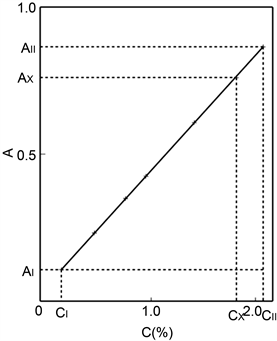# “精密度法则”在分光光度法中的作用The Role of “Precision Rule” in Spectrophotometry

DOI: 10.12677/AAC.2020.101001, PDF, HTML, XML, 下载: 376  浏览: 684

Abstract: Taking the determination of manganese in iron and steel as an example, this paper introduces the “precision rule”, i.e. “to maintain infection consistency of each factor for the each sample in the same measurement series”, which has two functions in photometry: 1) it makes the interference of various influencing factors on each sample in the same determination series tend to be the same, shows the true law of photometry, establishes the “two standard photometry”, establishes the theoretical basis for photometry to determine various contents, and changes the traditional concept; 2) It is the embodiment of normal distribution theory in photometry, which makes the measured values of samples approximate to normal distribution. Therefore, it is easy to find system errors and statistical outliers, to ensure the reliability of the measurement results, and to simplify the evaluation of uncertainty, to meet the requirements of JJF1059.1-2012 “Evaluation and Expression of Uncertainty in Measurement”.

1. 引言

2. 实验部分

2.1. 主要仪器及试剂

2.2. 实验方法

3. 结果与讨论

3.1. “精密度法则”在光度法中的作用

3.2. 化学物质吸光规律Table 1. Absorption of manganese standard sample at 520 nmFigure 1. “Calibration curve” of Manganese

$\left({A}_{x}-{A}_{I}\right)/\left({A}_{II}-{A}_{I}\right)=\left({C}_{x}-{C}_{I}\right)/\left({C}_{II}-{C}_{I}\right)$ (1)

${A}_{II}-{A}_{I}=k\left({C}_{II}-{C}_{I}\right)$ (2)

$\Delta A=k\Delta C$ (3)

“化学物质吸光规律”的存在，决定了入射光值域上、下限两个标准样品的坐标点连接线，即可作为样品含量的“测定线”，从而确立了“两标准光度法”，无需采用多个标准样品绘制“校准曲线”，因为“测定线”和“校准曲线”处于同一 $\Delta A=k\Delta C$ 的直线上。吸光度Ax对应的样品含量Cx，既可从“测定线”上查得，也可根据如下公式获得：

${C}_{x}=\left[\left({A}_{x}-{A}_{t}\right)\left({C}_{II}-{C}_{I}\right)/\left({A}_{II}-{A}_{I}\right)\right]+{C}_{I}$ (4)

${C}_{x}=\left[\left(0.614\right)\left(1.83\right)/\left(0.746\right)\right]+0.19=0.770+0.19=0.96\left(%\right)$

“化学物质吸光规律”不仅使光度法的实际操作得以简化，更重要的是，它奠定了光度法测定各种含量的理论基础，因为 $\Delta A=k\Delta C$ 中的含量改变量 $\Delta C$，既可是痕量的改变量，也可是高含量的改变量。

3.3. “精密度法则”是正态分布理论在光度法中的具体体现

3.4. “小样本”中系统误差的排除

3.5. “小样本”中统计离群值的排除

“小样本”测得值中常常存在异常数据，即统计离群值，它们会严重影响结果的可靠性。“小样本”近似正态分布，为发现统计离群值提供了条件。因为正态分布的样本均值 ${\stackrel{¯}{X}}^{\prime }$，会因测得值的不同而在极差中心的左右摆动，所以含最大残差ER的测得值，必然处于极差R与0.5R之间。我们采用“折半法”，在n = 5~10的“小样本”中，对其测得值进行选择，使超出( ${\stackrel{¯}{X}}^{\prime }±{E}_{R}$ )的测得值，即为GB/T4883-2008中格拉布斯法的“统计离群值” 。经过大量统计归纳，我们确定ER = 0.65R，即超出( ${\stackrel{¯}{X}}^{\prime }±0.65R$ )的测得值，就是应剔除的统计离群值。上述3.4中不含系统误差的9个测得值(%)：0.66、0.66、0.67、0.66、0.67、0.66、0.68、0.67、0.73， ${\stackrel{¯}{X}}^{\prime }$ = 0.67%，R = 0.07%。它们的( ${\stackrel{¯}{X}}^{\prime }±0.65R$ )区间：0.62%~0.72%，测得值0.73%超出了该区间，所以是个应当剔除的统计离群值。清除0.73%后 ${\stackrel{¯}{X}}^{\prime }$ = 0.67%既不含系统误差，也不含统计离群值，所以是可靠的。虽然清除0.73%前后的均值依然是0.67%，这并不表明这一清除没有必要，因为清除前的极差R = 0.07%，清除后的极差R = 0.02%。前者U95 = 0.02%，测定结果为(0.67 ± 0.02)%，kp = 2；后者U95 = 0.01%，测定结果为(0.67 ± 0.01)%，kp = 2。两个结果的不确定度差了一倍，表明只有排除了系统误差和统计离群值的结果才是真实可靠的。比如表2中“两标准光度法”锰、硅、磷的测得值，既排除了系统误差，同时又排除了统计离群值，所以它们才是真实可靠的 。Table 2. Determination results of “two standard photometry” for manganese, silicon and phosphorus

3.6. “增量光度法”的确立

3.7. 光度法测定结果的不确定度

$u\left({\stackrel{¯}{X}}^{\prime }\right)=S/\sqrt{n}$ (5)

${U}_{95}=2S/\sqrt{n},\text{}{k}_{p}=2$ (6)

${U}_{99}=3S/\sqrt{n},\text{}{k}_{p}=3$ (7)

S是样本观察值Xi的标准差。根据“规范”4.3.2.6之要求，“小样本”容量应取10。这时“小样本”必然近似正态分布，所以X10的S可按照极差法 $S=R/C$ 评估。n = 10，则 $C\approx 3$$S=R/3$。将 $S=R/3$ 代入式(6)、式(7)，即可得到不确定度的测量模型：

${U}_{95}=0.67R/\sqrt{n},\text{}{k}_{p}=2$ (8)

${U}_{99}=R/\sqrt{n},\text{}{k}_{p}=3$ (9)

3.8. 不确定度与允许误差的统一

${n}_{X}=n{\left({U}_{95}/\Delta \right)}^{2}\text{}\left(取整数\right)$ (10)

${n}_{X}=n{\left({U}_{99}/\Delta \right)}^{2}\text{}\left(取整数\right)$ (11)

4. 结语

  袁秉鉴. 高锰酸分光光度法的研究[J]. 化学分析计量, 2006, 15(2): 13-17.  袁秉鉴. 光度法测定不锈钢中的铬、镍、钼、钛、锰[J]. 化学分析计量, 2010, 19(1): 55-57.  尚庆坤, 崔运成, 赫春香, 等. 分析化学[M]. 北京: 科学出版社, 2014: 201-208.  彭晓文, 程玉红. 分析化学[M]. 北京: 中国铁道出版社, 2014: 126-130.  王彤, 段春生. 分析化学(第二版) [M]. 北京: 高等教育出版社, 2013: 237-244.  王玉枝, 张正奇, 宦双燕, 等. 分析化学(第三版) [M]. 北京: 科学出版社, 2016: 186-19.  王文渊, 曲中堂. 分析化学[M]. 北京: 化学工业出版社, 2013: 110-114.  王璇, 金涛, 王浩伟, 等. 紫外分光光度法测定聚硫密封胶中二氧化钛[J]. 化学分析计量, 2017, 26(4): 24-27.  高立红, 周凯红, 王燕霞, 等. 硅钼蓝分光光度法测定氟化稀土中二氧化硅[J]. 冶金分析, 2017, 37(4): 57-61.  邓军华, 王一凌, 亢德华, 等. 二安替吡啉甲烷光度法测定含钛物料中二氧化钛[J]. 冶金分析, 2015, 35(10): 30-35.  钟国秀, 黄清华. 光度法快速测定铜合金中微量铝[J]. 化学分析计量, 2011, 20(5): 60-62.  王冀艳, 刘勉, 赵晓亮, 等. 酸溶-苯基荧光酮光度法测定矿样中钽[J]. 冶金分析, 2017, 37(4): 48-51.  袁秉鉴, 任屏. 对光度法几点新认识[J]. 分析化学进展, 2019, 9(2): 132-138.  余君武, 肖艳清. 概率论与数理统计[M]. 北京: 理工大学出版社, 2009: 95.  杨振明. 概率论(第二版) [M]. 北京: 科学出版社, 2008: 74.  刘智敏. 误差与数据处理 [M]. 北京: 原子能出版社, 1981: 59-64.  袁秉鉴, 任屏. 两标准光度法测定钢铁中的锰、硅、磷[J]. 化学分析计量, 2017, 26(4): 71-74.  袁秉鉴. 光度法不确定度的数学模型[J]. 计量技术, 2010(1): 67-70.  GB/T4883-2008数据的统计处理和解释, 正态样本离群值的判断和处理[S].  袁秉鉴. 增量光度法测定巴士合金中的杂质铅[J]. 化学分析计量, 2004, 13(2): 20-22  JJF1059.1-2012测量不确定度评定与表示[S].  郑德如. 统计学[M]. 上海: 立信会计出版社, 1994: 173-177.  袁秉鉴. 重复测量结果的不确定度评定[J]. 中国计量, 2015(1): 89-90.  ISO/IEC17025 校准和测试实验室能力的通用要求[S].